# Least primitive root of prime numbers Least prime primitive root of prime numbers Least base necessary to prove the primality of a number

## Introduction

Let p be a prime number. Fermat's little theorem  states that a^(p-1) mod p=1 for all integers a between 1 and p-1. A primitive root  of p is a number r such that any integer a between 1 and p-1 can be expressed by a=r^k mod p, with k a nonnegative integer smaller that p-1. If p is an odd prime number then r is a primitive root of p if and only if r^((p-1)/q) mod p>1 for all prime divisors q of p-1. If a number r can be found that satisfies these conditions, then p must be a prime number. In fact, it is possible to relax the above conditions in order to prove that p is prime ; it is sufficient to find numbers r_k such that (r_k)^((p-1)/q_k) mod p>1 and (r_k)^(p-1) mod p=1 for all prime divisors q_k of p-1 (these conditions guarantee the existence of a primitive root of p).

A famous conjecture of Emil Artin [3, problem F9],  states that if a is an integer other than -1 or a perfect square, then the number N(x;a) of primes p <= x such that a is a primitive root mod p is given asymptotically by A(a) pi(x) for some positive constant A(a), where pi(x) is the usual prime counting function. Furthermore, the values of A(a) are rational multiples of the constant

```             /        1    \
A = PRODUCT  | 1 - ------- | = 0.37395581361920228805...
p prime  \     p (p-1) /
```

called, appropriately, Artin's Constant. In this table [5KiB, compressed with gzip] we present the values of A(a)/A for all a up to 1000.

The Artin conjecture has been generalized in the following way : let N(x;a_1,...,a_n) be the number of primes p <= x such that a_1,...,a_n are simultaneously primitive roots mod p. The generalized Artin conjecture states that N(x;a_1,...,a_n) is given asymptotically by A(a_1,...,a_n) pi(x) for some non-negative constant A(a_1,...,a_n). Some of these constants are zero; for example, A(2,3,6)=0 because 6 cannot be a primitive root if 2 and 3 are primitive roots. A complicated formula for A(a_1,...,a_n) is given in .

Let g(p) and G(p) denote, respectively, the least primitive root and the least prime primitive root of the prime number p. It is not difficult to verify that g(p) cannot be a perfect power. Also, let B(p) denote the least prime base required to prove the primality of p using the test mentioned above, when the bases r_k used in this test are restricted to be prime numbers. Let Ng(x;r) be the number of primes p <= x such that g(p)=r. Define likewise NG(x;r) and NB(x;r). Using the inclusion-exclusion principle applied to the Matthews' generalized Artin conjecture, it is expected that the ratios Ng(x;r) / pi(x), NG(x;r) / pi(x), and NB(x;r) / pi(x) approach constants when x goes to infinity. In particular, Ng(x;2) / pi(x) should converge to Artin's constant.

Let qg(n) be the smallest prime number q for which g(q)=n, with n not a perfect power, and let qG(p) and qB(p) be defined in a similar way, with p a prime number. The values of these functions for each admissible n or p are also of theoretical interest, in particular in what concerns their rate of growth.

## Computational results (April 29, 2001)

We have implemented a program that computes the values of g(p), G(p), and B(p) for each prime number p in a given interval. Our program records the number of times each one of those values occurred, as well as the value of p for which they first occur. Each run of our program tests an interval of 10^10 integers, and takes, on a 400MHz Pentium Celeron processor, around seven hours to finish. We have used a modified segmented sieve of Eratosthenes to record, for each integer, its largest factor not larger than its square root. This makes the primality test trivial, and speeds up considerably the factorization of the (even) numbers p-1. The least primitive root computation is done in assembly language, to take advantage of some floating point capabilities of the processor (this alone resulted in a very significant speedup of our program). The core of the program was compiled (into an object file) on a Linux machine, and linked with encapsulation code required to make the program a Windows NT service. A Linux-only version is also in use. The program uses sockets to communicate with a central server, which manages the entire computation. This program was run for some time on the spare time of the computers of a classroom of the Electronics and Telecommunications Department of the University of Aveiro, as well as on the spare time of the computers of some friends, viz., António Teixeira, Armando Pinho, Carlos Bastos, Joaquim Sousa Pinto, Luis Silva, and Miguel Oliveira e Silva. In April 2001 the limit 10^14 was reached and double-checked.

More recently (2014-2015), we have made several improvements to the program. We now have some results about least positive or negative primitive roots and least positive or negative prime primitive roots up to 10^16 (preliminary data [10KiB, compressed with gzip]).

With the old program we have tested, and double-checked, all prime numbers up to 10^14. (As far as we are aware, the previous record of computation was 35·10^9 , .) We present below a summary of our results for least primitive roots, least prime primitive roots, least prime base required to prove the primality of a number, as well as empirical estimates of the Artin constant and of the average value of the least (prime) primitive root.

### Least primitive roots

In this table [3KiB, compressed with gzip] we present the first occurrences of the values of g(p) we were able to compute, i.e., values of qg(n), as well as counts of the number of times each g(p)=n occurred, i.e., values of Ng(x;n). The record-holders, i.e., numbers larger than all previous ones of the same kind, are clearly marked in the table. The following two figures present graphs with the available values of qg(n) and of Ng(x;n) for our old interval of computation.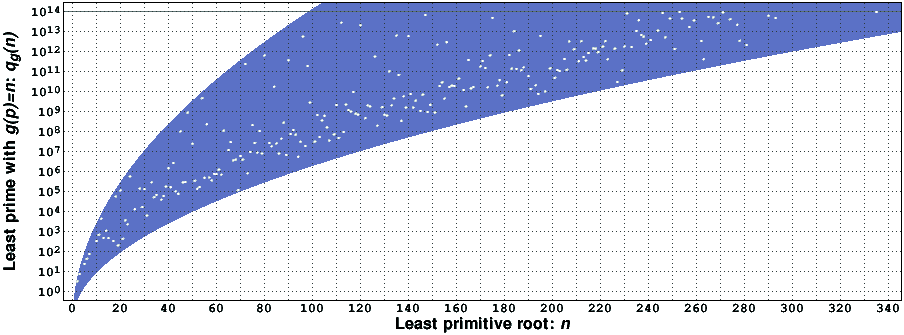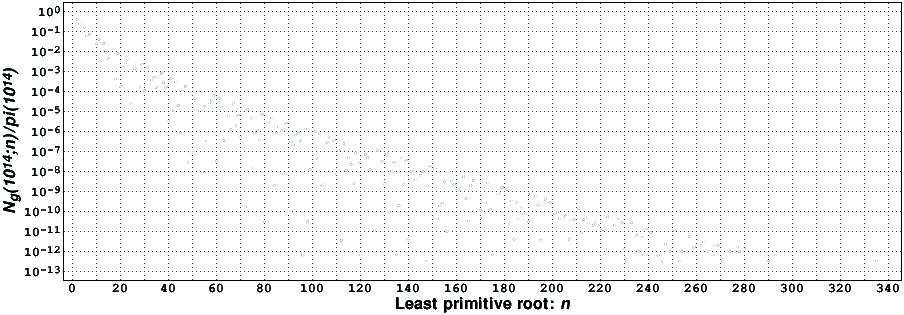We have observed empirically that

```        1.8 sqrt(n)                       3.6 sqrt(n)
0.03  e             <  q_g(n)  <  0.03  e            .
```

(The region between these two bounds is clearly marked in the appropriate figure.) It is interesting to observe that the upper bound appears to be close to the square of the lower bound. From this empirical lower bound, after rounding some numbers, we obtain g(p)<0.3(4+log p)^2, which gives a reasonably tight empirical upper bound for g(p).

### Least prime primitive roots

In this table [2KiB, compressed with gzip] we present the first occurrences of the values of G(p) we were able to compute, i.e., values of qG(p), as well as counts of the number of times each G(q)=p occurred, i.e., values of NG(x;p). The record-holders are, as usual, clearly marked in the table. The following two figures present graphs with the available values of qG(p) and of NG(x;p) for our old interval of computation.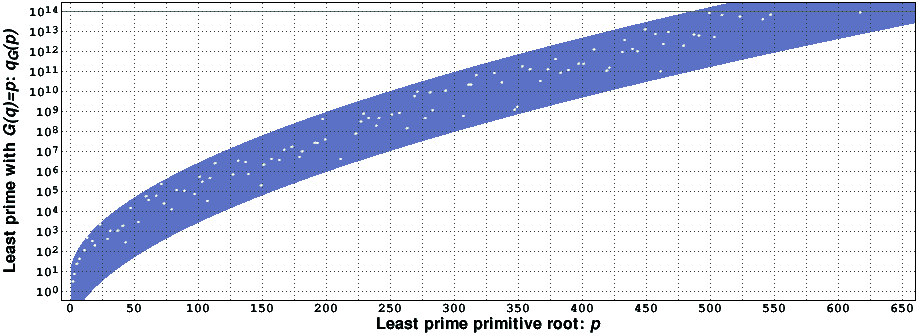This last graph becomes much more regular if instead of using the primes pk in the x axis, with p1=2, p2=3, etc., one just uses their index (i.e., only k). We also have observed empirically that

```         0.55                    0.55
p                       p
0.01  e       <  q_G(p)  <  9 e      .
```

(The region between these two bounds is clearly marked in the appropriate figure.) In this case, it appears that the two bounds grow in the same way.

### Least prime base required to prove the primality of a number

In this table [1k, compressed with gzip] we present the first occurrences of the values of B(p) we were able to compute, i.e., values of qB(p), as well as counts of the number of times each B(q)=p occurred, i.e., values of NB(x;p). The record-holders are, as usual, clearly marked in the table. The following two figures present graphs with the available values of qB(p) and of NB(x;p) for our old interval of computation.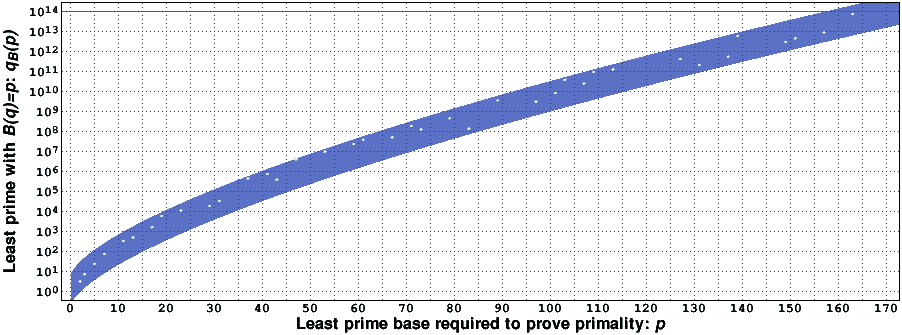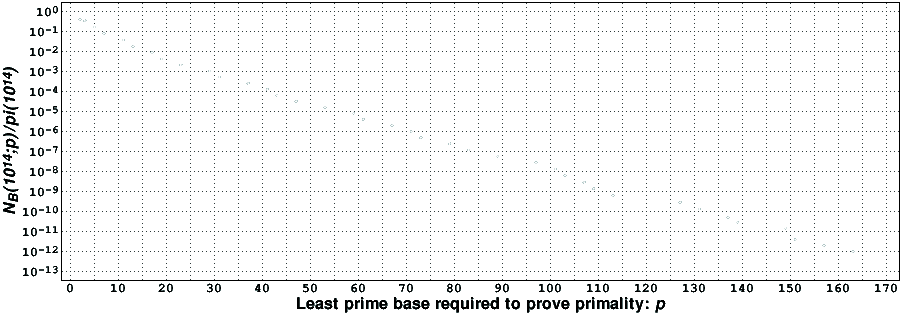Like in the least prime primitive roots case, this last graph becomes more regular if instead of using the primes pk in the x axis one just uses their index. We also have observed empirically that

```        0.675                   0.675
p                       p
0.2  e       <  q_B(p)  <  6 e      .
```

(The region between these two bounds is clearly marked in the appropriate figure.) It appears that the two bounds also grow in the same way.

### Artin' constant

To continue the preliminary analysis of our computational results, in this table [2k, compressed with gzip] we present the number of odd primes in the intervals [n·10^12,(n+1)·10^12], n=0,...,99, together with the number of odd primes in these intervals for which 2 is a primitive root. Dividing the latter by the former we obtain estimates of the Artin constant, which are presented in the following figure.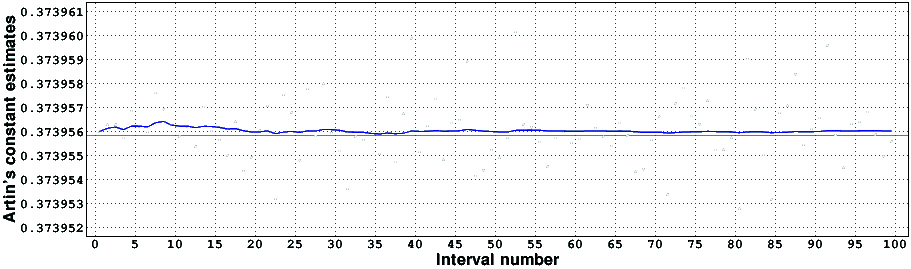The white dots are the estimates for each individual interval; they appear to be uncorrelated. The blue line are the estimates using all the data available up to that point. The thin blue line is the value of the Artin constant. The value of this constant, computed using analytic means , , is 0.3739558136..., and should be compared with 0.3739559970 (the last few digits are probably wrong), which is our most precise estimate. The difference between the two is quite small, and is close to the inverse of the square root of the number of primes in the test interval. Thus, we may say that there is a good agreement between what the theory predicts (under some unproven hypotheses) and what the numerical computations actually reveal.

### Average value of the least (prime) primitive root

To conclude the preliminary analysis of our computational results, in this table [3k, compressed with gzip] we present the number of odd primes in the intervals [n·10^12,(n+1)·10^12], n=0,...,99, together with the values of the sums of the values of g(p), G(p), and B(p), for all odd primes p inside each of these intervals. From this data it is possible to estimate the average values of g(p) and of G(p), which, as suggested in , should be finite. The following figure presents our estimates of the average value of g(p). The estimates of the average values of G(p) and of B(p) give rise to similar-looking figures.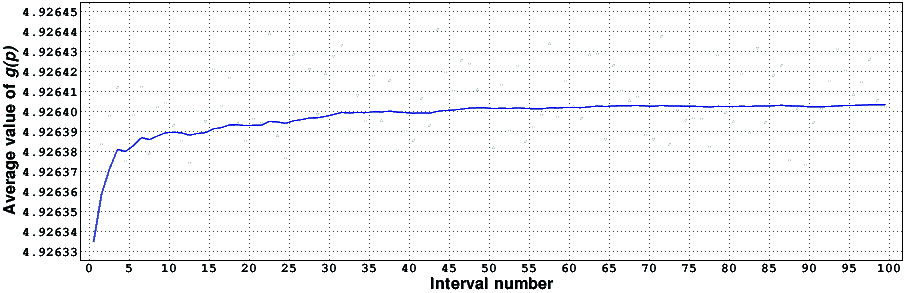The white dots are the estimates for each individual interval. The blue line are the estimates using the data of all previous intervals. It appears that the estimated average values of g(p) are indeed converging, albeit at a somewhat slow rate. Using all available data, our estimates of the average values of g(p), G(p), and B(p) are, respectively, 4.926403, 5.908773, and 3.974831 (the last one or two digits of these estimates are probably wrong).

## References

  Paulo Ribenboim, The new book of prime number records, Springer, 1995.  Hans Riesel, Prime numbers and computer methods for factorization, second edition, Birkhäuser, 1994.  Richard K. Guy, Unsolved problems in number theory, third edition, Springer-Verlag, 2004.  K. R. Matthews, A generalization of Artin's conjecture for primitive roots, Acta Arithmetica, vol. XXIX, pp. 113-146, 1976.  Andrzej Paszkiewicz, Letter dated August 12, 1999.  P. D. T. A. Elliott and Leo Murata, On the average of the least primitive root modulo p, Journal of the London Mathematical Society, vol. 56, no. 2, pp. 435-454, 1997.  Pieter Moree, Approximation of singular series and automata, Manuscripta Mathematica, vol. 101, pp. 385-399, 2000.  Eric Bach, The complexity of number-theoretic constants, Information Processing Letters, vol. 62, pp. 145-152, 1997.